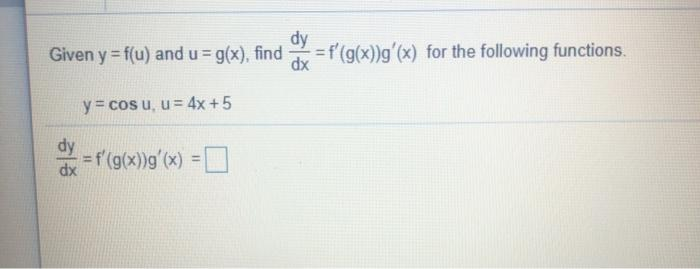### Create an Account

Home / Questions / dy Given y = f(u) and u = g(x), find = f'(g(x))g'(x) for the following functions. dx y = c...

# dy Given y = f(u) and u = g(x), find = f'(g(x))g'(x) for the following functions. dx y = cosu, u = 4x +5 dy = f'(g(x))g'(x) x(= dx

dy Given y = f(u) and u = g(x), find = f'(g(x))g'(x) for the following functions. dx y = cosu, u = 4x +5 dy = f'(g(x))g'(x) x(= dxMay 04 2021 View more View LessSubscribe To Get Solution